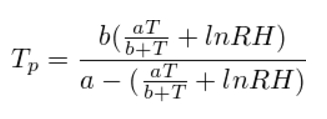# Bubble-point test

This calculator has a continuation of the previous calculator for determining the humidity of the air with the help of psychrometric method.

Dewpoint - t0C, to which air must be cooled to water vapor (which is in the air) has reached its saturation limit (gas humidity is 100%). Then, when the air cools, condensate is formed. When positive T0c - mildew, and if the red - frost, ice or snow.

A small example. In a sufficiently warm environment brought some thing from the street (meaning from the cold). The air around the surface of the still cold, things cooled below the dew point (for a given humidity and t0C) and on the surface of condensation is released. Then the thing heats up t0C premises, and the condensation has evaporated.

dew point calculation can be written as a formula here:where a = the exact number 17.27, b = exact number of 237.7, lnRH - logarithm of relative humidity (measured in fractions of unity), Tp - dew point respectively.

According to Wikipedia, in the range of 0 to 60 0C formula is 0.4 0C error

﻿

Definition point dew
Point dew (C):